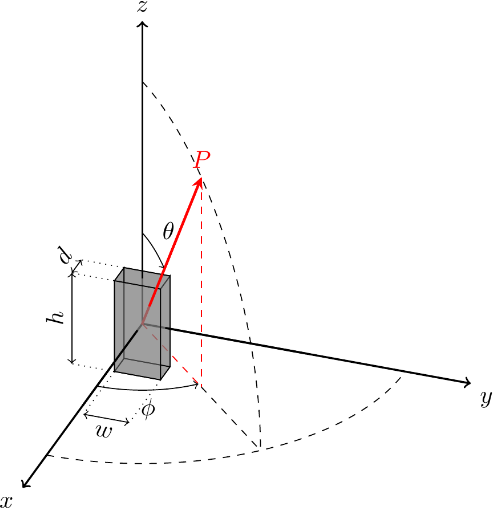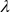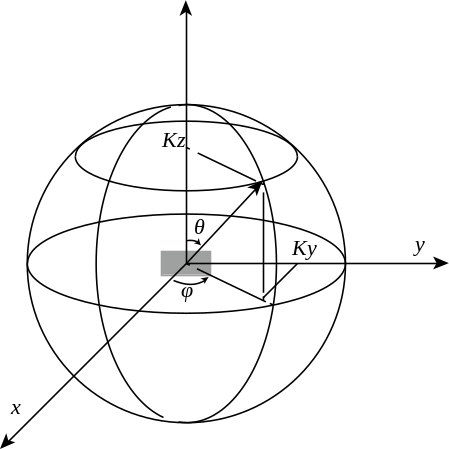## F.2 Spherical equal angle grid

37.145-23GPPActive Antenna System (AAS) Base Station (BS) conformance testingPart 2: radiated conformance testingRelease 17TS

## F.2.1 General

TRPEstimate is defined as:

when EIRP measurements is used or as:

when power density measurements are used, and d is the test distance. N and M are the number of samples in the and angles. Each () is a sampling point. The sampling angular intervals for and angles are and . The sampling intervals and are described in F.2.2.

## F.2.2 Reference angular step criteria

The reference angular steps and , in degrees, are defined as:

The upper limit for these reference angular steps of ensures a low Summation Error (SE) when is large compared to the DUT dimensions.

Dcyl and D are calculated as:

The definition of d, w and h is shown in Figure F.2.2-1. The radiation source can be EUT antenna array or the whole of EUT.Figure F.2.2-1: Dimensions of a radiation source: depth (d), width (w) and height (h)

Optionally, in the case of a Uniform Linear Array (ULA), when d is negligible (d ) and when the EUT is mounted along the yz plane as shown in figure F.2.2-2, the reference angular step, in degrees, can be determined by

Where Dy is the length of radiating parts of EUT along y-axis, Dz is the length of radiating parts of EUTalong the z-axis andis wavelength for the measured frequency.Figure F.2.2-2: Spherical coordinate for OTA conformance testing of EUT

Where due to practical reasons such as time constraints or turn-table precision, measurement with the reference steps is not practical, sparser grids can be used. Use of sparse grids can lead to errors in TRP assessment. In order to characterize these errors, the SF (sparsity factor) of the grid is defined as:

Where and are the actual angular steps used in the measurement.

Alternatively, when the EUT radiating dimensions are not known. For each frequency within the downlink operating band including ΔfOBUE, the reference angular steps can be specified in terms of the beamwidth of the wanted signal as

where λo is the wavelength of the wanted signal, and BeWφ and BeWθ are the beamwidth of the wanted signal in the φ-axis and θ-axis, respectively.

BeWφ and BeWθ may be set to beamwidth declared for the OTA AAS BS radiated transmit power requirement provided the same beam is applied to test in-band TRP requirements.

NOTE: Beamwidth is approximately equal to half the first-null beam width.